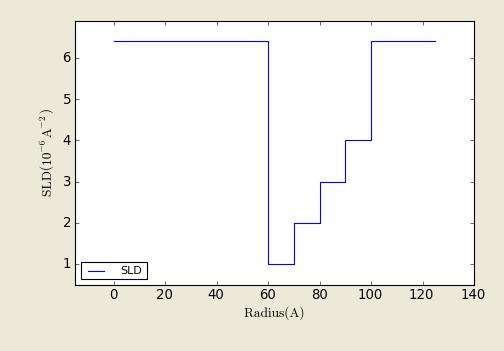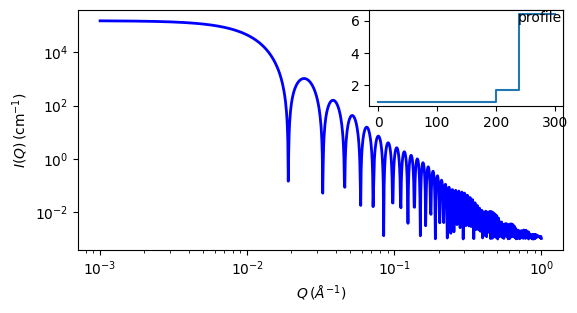# core_multi_shell

This model provides the scattering from a spherical core with 1 to 10 concentric shell structures. The SLDs of the core and each shell are individually specified.

Parameter

Description

Units

Default value

scale

Scale factor or Volume fraction

None

1

background

Source background

cm-1

0.001

sld_core

Core scattering length density

10-6-2

1

200

sld_solvent

Solvent scattering length density

10-6-2

6.4

n

number of shells

None

1

sld[n]

scattering length density of shell k

10-6-2

1.7

thickness[n]

Thickness of shell k

40

The returned value is scaled to units of cm-1 sr-1, absolute scale.

Definition

This model is a trivial extension of the CoreShell function to a larger number of shells. The scattering length density profile for the default sld values (w/ 4 shells).Fig. 77 SLD profile of the core_multi_shell object from the center of sphere out for the default SLDs.*

The 2D scattering intensity is the same as $$P(q)$$ above, regardless of the orientation of the $$\vec q$$ vector which is defined as

$q = \sqrt{q_x^2 + q_y^2}$

Note

Be careful! The SLDs and scale can be highly correlated. Hold as many of these parameters fixed as possible.

Note

The outer most radius (= radius + thickness) is used as the effective radius for $$S(Q)$$ when $$P(Q)*S(Q)$$ is applied.

For information about polarised and magnetic scattering, see the Polarisation/Magnetic Scattering documentation.

Our model uses the form factor calculations implemented in a C-library provided by the NIST Center for Neutron Research .Fig. 78 1D plot corresponding to the default parameters of the model.

Source

core_multi_shell.py $$\ \star\$$ core_multi_shell.c $$\ \star\$$ sas_3j1x_x.c

References

Also see the core_shell_sphere model documentation and 

Authorship and Verification

• Author: NIST IGOR/DANSE Date: pre 2010